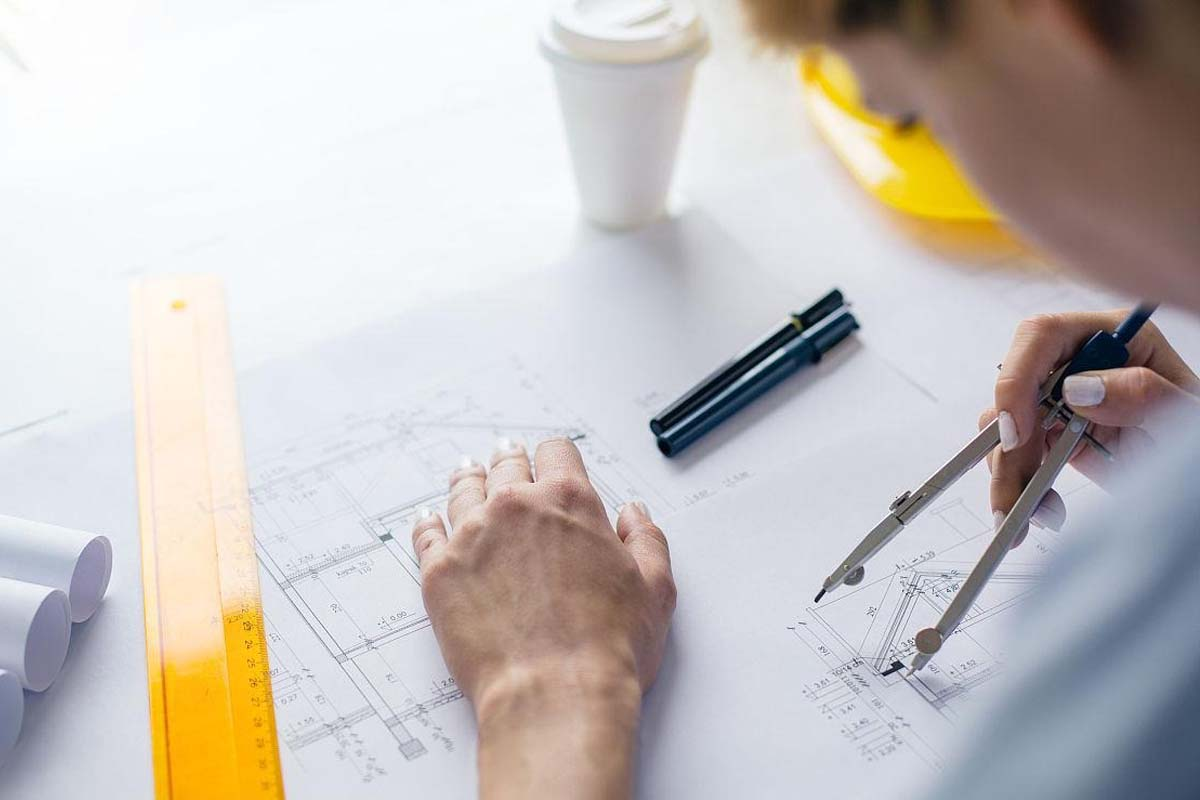## Structural Steel Pipe Brand Leader

Language:
Home / International Common Conversion Table

# International Common Conversion Table

INTERNATIONAL COMMON UNIT CONVERSION TABLEAT YUANTAI DERUN IT DOESN’T MATTER IF YOU NEED NON-STANDARD SIZES OR YOU HAVE AN UNUSUAL REQUEST. WITH OUR EXPERTISE AND RANGE OF STOCK, WE CAN MEET ALL YOUR STRUCTRURAL STEEL  PIPE AND STEEL PROFILE REQUIREMENTS.
Length
1 inch = 2.540 centimeters
1 inch = 25.4 millimeter
1 meter = 100 centimeters
1 meter = 1000 millimeters
1 centimeter = 10 millimeters
1 millimeter = 0.03937 inches
1 meter = 10 decimeters
1 decimeters = 10 centimeters
1 foot = 30.4878 centimeters
1 centimeters = 0.3937 inches
1 yard = 0.9144028 meters
1 meter = 3.281 feet
1 mile = 1.6093419 kilometer
1 kilometer = 0.621372 miles
Area
1 sq. meter = 100 sq. decimeters
1 sq.decimeter = 100 sq. centimeters
1 sq.centimeter = 100 sq.millimeters
1 sq. meter = 1550.00310001 sq. inches
1 sq. inch = 6.4516 sq. centimeters
1 sq. centimeter = 0.1550 sq. inches
1 sq. foot = 0.0929 sq. meters
1 sq. meter = 1.195986 sq. yards
1 sq. yard = 0.83613 sq. meters
1 sq. kilometer = 0.386101 sq. miles
1 sq. mile = 2.589999 sq. kilometers
1 hectare = 2.471044 acres
1 acre = 0.404687 hectares
Mass (Weight)
1 grain = 0.05 scruples = 0.016667 drams = 0.00208 ounces
1 ounce = 28.3495 grams
1 gram = 0.03527396 ounces
1 pound = 16 oz = 0.4535924 kilograms
1 kilogram = 2.2046223 pounds
1 short ton = 0.892857 metric ton
1 metric ton = 1.1200 short tons
1 long ton = 1.01605 metric tons
1 kilometer = 0.621372 miles
Volume
1 liter（L）=1000 milliliters（ML）
1 liter（L）=10 deciliters（DL）
1 teaspoon = 5 milliliters
1 liter（L）=0.001 cubic meters (m³)
1 milliliter = 0.0338147 fluid ounces
1 tablespoon = 15 milliliters
1 liter = 2.11342 pints = 1000 cubic centimeters
1 fluid ounce = 30 milliliters
1 liter = 1.05671 quarts = 0.264178 gallons
1 gallon = 3.785332 liters = 231 cubic inches
1 cup = 0.23658 liters
1 pint = 0.473167 liters
1 cubic meter = 35.3144 cubic feet = 1.30794 cubic yards
1 cubic foot = 0.0283170 cubic meters
1 cubic yard = 0.764559 cubic meters
Temperature
Fahrenheit Subtract 32, then multiply by 5/9ths Celsius
Celsius Multiply by 9/5ths, then add 32 Fahrenheit

Formula for calculating the weight of steel pipes per meter

Formula for calculating the weight of square tubes:

4 × wall thickness × (Side length wall thickness) × 7.85/1000 kg/m

For example, 50 × 50 ×  4mm square tube weight is: 4 × 4 × (50-4) × 7.85/1000 kg/m=5.77 kg/m

Formula for calculating the weight of rectangular tubes:

2 × (Side length 1+Side length 2- Wall thickness) × wall thickness × length × Density kg/m/1000

For example, 60 × 40 ×  4mm rectangular tube weight is:

2 × (60+40-4) × 4 × 1 × 7.85 kg/m/1000=6.0288 kg/m

Usually, this calculation company calculates a weight that is greater than the actual weight.

There is also a calculation formula for square and rectangular steel pipes:

There is also a formula for calculating the weight per meter for square and rectangular steel pipes:

（2 × (Side length 1+Side length 2)/3.14- Wall thickness) ×  Wall thickness ×  0.0246615 ×  Length/1000

Formula for calculating the weight of circular pipes:

(Outer diameter - wall thickness) ×  Wall thickness × 0.0246615 ×  Length/1000

For example, 2032 ×  40mm round tube weight is:
(2032-40)×40×0.0246615×1/1000=1.96502832 Ton/M

Density
Grey cast iron (≤ HT200) 7.2 （g/cm ³）
Grey cast iron (≥ HT250) 7.35 （g/cm ³）
White cast iron 7.4~7.7 （g/cm ³）
Malleable cast iron 7.2-7.4 （g/cm ³）
Industrial pure iron 7.87 （g/cm ³）
Cast steel 7.8 （g/cm ³）
Steel 7.85 （g/cm ³）
Low carbon steel (containing 0.1% carbon) 7.85 （g/cm ³）
Medium carbon steel (containing 0.4% carbon) 7.82 （g/cm ³）
High carbon steel (containing 1% carbon) 7.81 （g/cm ³）
High speed steel (containing 9% tungsten) 8.3 （g/cm ³）
High speed steel (containing 18% tungsten) 8.7 （g/cm ³）
Stainless steel (1Cr18Ni9) 7.93 （g/cm ³）
Stainless steel (1Cr13) 7.75 （g/cm ³）
Stainless steel (1Cr17) 7.70 （g/cm ³）
Pure copper (red copper) 8.93 （g/cm ³）
Pure nickel, NSi0.19 8.902 （g/cm ³）
Industrial pure aluminum 2.702 （g/cm ³）
Zinc plate, zinc anode plate 7.133 （g/cm ³）
Zinc copper alloy 7.2 （g/cm ³）
Tin 7.168 （g/cm ³）
Osmium 22.59 （g/cm ³）
Iridium 22.56 （g/cm ³）
Platinum 21.45 （g/cm ³）
Jin 19.32 （g/cm ³）
Tungsten 19.35 （g/cm ³）
Tantalum 16.6 （g/cm ³）
Mercury 13.546 （g/cm ³）
Thorium 11.72 （g/cm ³）
Silver 10.5020 （g/cm ³）
Molybdenum 10.22 （g/cm ³）
Bismuth 9.808 （g/cm ³）
Cobalt 8.832 （g/cm ³）
Niobium 8.57 （g/cm ³）
Manganese 7.47 （g/cm ³）
Chromium 7.19 （g/cm ³）
Cerium 6.6893 （g/cm ³）
Antimony 6.697 （g/cm ³）
Zirconium 4.574 （g/cm ³）
Tellurium 6.237 （g/cm ³）
Titanium 4.507 （g/cm ³）
Barium 3.512 （g/cm ³）
Beryllium 1.8520 （g/cm ³）
Magnesium 1.738 （g/cm ³）
Calcium 1.55 （g/cm ³）
Sodium 0.9674 （g/cm ³）
Potassium 0.862 （g/cm ³）
Arsenic 5.727 （g/cm ³）
Selenium 4.809 （g/cm ³）
Boron 2.3420 （g/cm ³）
Silicon 2.33 （g/cm ³）
Disclaimer: While we have made efforts to ensure the accuracy of the conversions and formulas on this website, we are not liable for any inaccuracies. We do not guarantee the accuracy, reliability, or completeness of the information provided here. Visitors acknowledge and agree that any reliance on this website's information is at their own risk. yuantaisteelpipe.com shall not be liable for any damages resulting from the use or performance of this website.